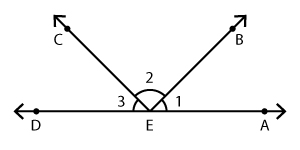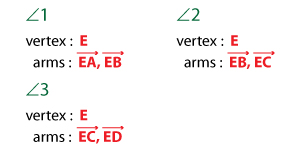# Parts of an Angle | Vertex and Arms of an Angle

1. Math Lessons >
2. Parts of an Angle

When two rays meet at a common endpoint, an angle is formed.

An angle has two major parts.

Vertex: The common endpoint.

Arms: The two rays that make an angle.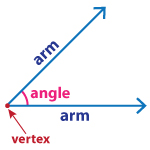Naming the vertex and arms of an angle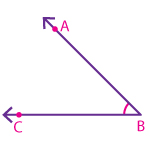Here, B is the vertex of the angle;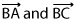are the two arms of the angle.

Want to go after it right away? Here's a little practice.

1. Take a closer look at the image, and find out the vertex and arms of each angle.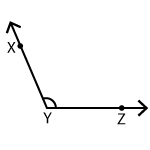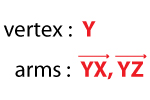2. It's time to take your learning a notch up! Draw an angle with the vertex Q and arms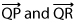.Take up the challenge!

Name the vertex and arms that form each angle in the given figure.